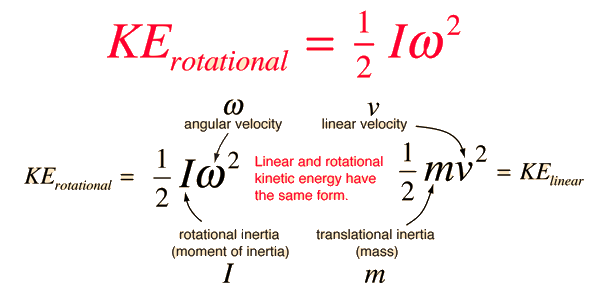# Rotational Kinetic Energy

The kinetic energy of a rotating object is analogous to linear kinetic energy and can be expressed in terms of the moment of inertia and angular velocity. The total kinetic energy of an extended object can be expressed as the sum of the translational kinetic energy of the center of mass and the rotational kinetic energy about the center of mass. For a given fixed axis of rotation, the rotational kinetic energy can be expressed in the formThe expressions for rotational and linear kinetic energy can be developed in a parallel manner from the work-energy principle. Consider the following parallel between a constant torque exerted on a flywheel with moment of inertia I and a constant force exerted on a mass m, both starting from rest.For the linear case, starting from rest, the acceleration from Newton's second law is equal to the final velocity divided by the time and the average velocity is half the final velocity, showing that the work done on the block gives it a kinetic energy equal to the work done. For the rotational case, also starting from rest, the rotational work is τθ and the angular acceleration α given to the flywheel is obtained from Newton's second law for rotation. The angular acceleration is equal to the final angular velocity divided by the time and the average angular velocity is equal to half the final angular velocity. It follows that the rotational kinetic energy given to the flywheel is equal to the work done by the torque.

 Rolling objects
Index

Moment of inertia concepts

Rotational kinetic energy concepts

 HyperPhysics***** Mechanics ***** Rotation R Nave
Go Back